## Example Questions

← Previous 1

### Example Question #1 : How To Find The Percent Of Increase

A manager of a pizzeria has five people on his staff. Three are full-time staff, each of whom received a starting salary of $25,000 annually for 40 hours of work per week. Each year they have worked at the pizzeria, their pay increases by 7%. Sally has worked at the pizzeria for one year, Nancy for two years and John for three years. The other two employees are part-time and are paid$8.25 per hour. Irene works 1,000 hours per year and Andrew works 700 hours per year. What is the pizzeria’s cost per year for staff salaries?

Possible Answers:

$94,397.50$84,275

$96,872.50$86,025

$92,525.50 Correct answer:$94,397.50

Explanation:

It is important to remember that the employees who receive an annual increase (Sally, Nancy and John), receive the 7% increase based on the salary from the year before.

### Example Question #3 : How To Find The Percent Of Increase

Truck A has a length of 8'3" (8 ft. and 3 inches).  Truck B has a length of 14'5".

What is the percentage increase in length going from Truck A to Truck B?

Possible Answers:

66.53

74.74

35.42

79.11

Correct answer:

74.74

Explanation:

Find a common unit—in this case it is inches. The increase is from 99” to 173”.  To find the percentage increase we find the difference and then divide by the length of Truck A.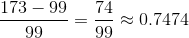or 74.74%

### Example Question #4 : How To Find The Percent Of Increase

Danielle has two jobs.  Her retail job pays her $8.50 per hour and she works 20 hours per week in that job. Her office job pays her$12 per hour and she works 15 hours per week in the office.  If she starts working an extra 10 hours a week at her office job, what is her percentage increase in total weekly pay? Round to one decimal point.

Possible Answers:

33.4%

34.3%

40.0%

25.7%

22.9%

Correct answer:

34.3%

Explanation:

Pay = Hours worked x Rate per hour

Retail job = 20 x $8.50 =$170.00

Office job = 15 x $12.00 =$180.00

Current weekly pay = $170.00 +$180.00 = $350.00 Additional Office job hours = 10 x$12.00 = $120.00 Percentage Increase =$120 / \$350 = .343 (rounded) --> 34.3%

### Example Question #5 : How To Find The Percent Of Increase

A university has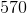students currently enrolled in its freshman class. Last year, there were only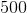freshman enrolled at the school. By what percentage did the number of students in the freshman class increase?

Possible Answers: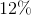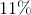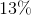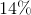Correct answer:Explanation:

To find the percentage increase, use the following formula:

Increased # of students this year / # of students last year

If there werestudents last year, and this year there are, the number of students increased by.  Then, knowing this, you can find what percentage of the original number ()represents.

Therefore: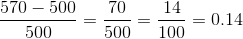The number of students increased by.

### Example Question #6 : How To Find The Percent Of Increase

Thurman is given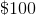. Each day, he gains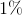on this dollar amount, then losesof his total each night. If Thurman gains no other money, what will eventually happen to the total amount of money?

Possible Answers:

It will decrease over time.

It will increase linearly over time.

It will increase exponentially over time.

It will remain the same over time.

It will first decrease, then increase.

Correct answer:

It will decrease over time.

Explanation:

To find out which option is correct, simply follow one complete cycle (that is to say, one day's worth of actions).

We will useto represent the initial amount.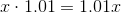First, increase by.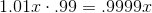Next, decrease by.

So, after each cycle, the total amount of money decreases by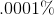. Eventually therefore, the money will run out.

### Example Question #7 : How To Find The Percent Of Increase

On a given day, the temperature ranges from a low of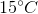to a high of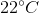. What is the percent increase of temperature from the day's low to high temperature? Round to the nearest integer.

Possible Answers: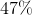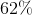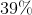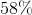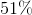Correct answer:Explanation:

To find percent change, subtract the "old" amount from the "new" amount, then divide the result by the "old" amount. The result is a percent increase if positive and a percent decrease if negative.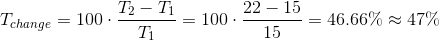Thus, there was aincrease in temperature that day.

### Example Question #8 : How To Find The Percent Of Increase

What is the percent increase from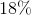of a number toof that same number?

Possible Answers: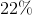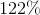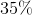Correct answer:Explanation:

To find percent change, subtract the "old" amount from the "new" amount, then divide the result by the "old" amount. The result is a percent increase if positive and a percent decrease if negative. In this case, don't forget: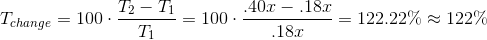### Example Question #9 : How To Find The Percent Of Increase

The price of a particular metal raises from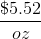to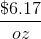. What percent increase did the price see?

Possible Answers: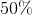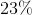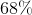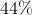Correct answer:Explanation:

To find percent change, subtract the "old" amount from the "new" amount, then divide the result by the "old" amount. The result is a percent increase if positive and a percent decrease if negative.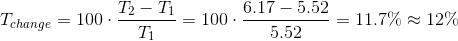### Example Question #10 : How To Find The Percent Of Increase

A particular stock gainson its price on Tuesday. On Wednesday, it losesof its new price. On Thursday, it gains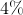on Wednesday's price. What is the percent increase at the close of business Thursday, compared to the opening price on Tuesday? Do not round until the final answer.

Possible Answers: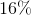Correct answer:Explanation:

To find percent change, subtract the "old" amount from the "new" amount, then divide the result by the "old" amount. The result is a percent increase if positive and a percent decrease if negative. In this case, to keep our amount constantly compared to Tuesday, set Tuesday's price as a variable.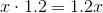On Tuesday, gain 20 percent.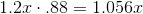On Wednesday, lose 12 percent (thus, multiply by 88 percent).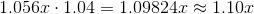On Thursday, gain 4 percent.

Thus, our final gain relative to Tuesday is.

← Previous 1

### All ACT Math Resources